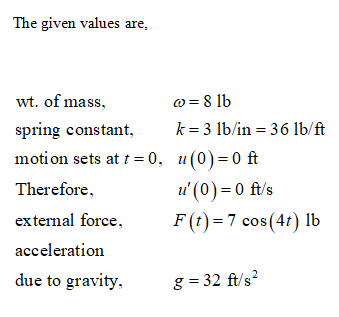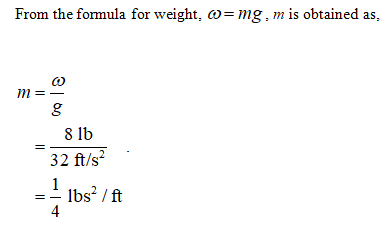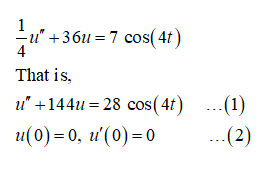# An undamped spring-mass system contains a mass that weighs 8lb and a spring with spring constant 3lb/in. It is suddenly set in motion at t=0 by an external force of 7cos⁡(4t)lb. Determine the position u, in feet, of the mass at any time t. Use 32ft/s2 as the acceleration due to gravity. Pay close attention to the units.u(t)  = ? ft

Question
93 views

An undamped spring-mass system contains a mass that weighs 8lb and a spring with spring constant 3lb/in. It is suddenly set in motion at t=0 by an external force of 7cos⁡(4t)lb.

Determine the position u, in feet, of the mass at any time t. Use 32ft/s2 as the acceleration due to gravity. Pay close attention to the units.

u(t)  = ? ft

check_circle

Step 1Step 2Step 3

Therefore, the given initial value problem is described as follows....

### Want to see the full answer?

See Solution

#### Want to see this answer and more?

Solutions are written by subject experts who are available 24/7. Questions are typically answered within 1 hour.*

See Solution
*Response times may vary by subject and question.
Tagged in